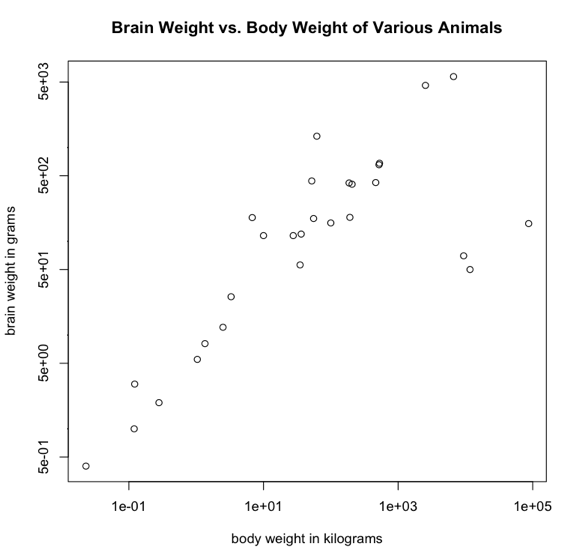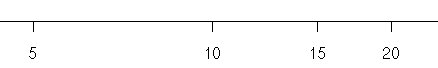Logarithms turn a geometric (or multiplicative) sequence of numbers into an additive one. Thus, it compresses the sequence with the greatest compression effect being at the larger values. This is illustrated by the following sequence, which has its base 10 logs shown below it. ``` sequence 1 10 100 1000 10000 100000 1000000 logs base 10 0 1 2 3 4 5 6``` In the sequence, each successive value is the previous value multiplied by 10, a geometric (or multiplicative) sequence. Taking the logs changes this to a sequence in which each value is the previous value plus 1, an additive sequence. The definition of base 10 logs is: log10 N = x if 10x = N (Remember Ben? BE = N. Based to an exponent equals number.) Thus, log10 10000 = 4 because 104 = 10000. In scientific work, base 10 logs are rarely used any more. Natural logs have taken their place. In natural logs, the base is 2.718281828..., which is the mathematical constant e. Thus, these are sometimes also called "base e logs." Here are some useful rules for logarithms that you will need to remember. log(xy) = log(x) + log(y) log(xa) = a log(x) loga(a) = 1, e.g., loge(e) = 1, log10(10) = 1, etc. log(x/y) = log(x) - log(y) elog(x) = x (assuming base e logs) exp(x) = ex (thus, exp is the antilog function for natural logs) log(1) = 0 logs of numbers between 0 and 1 are negative, and logs of numbers less than or equal to 0 do not exist In statistics, log transforms (i.e., taking the log of every value of a variable) are often used to make a positively skewed distribution more normal, or to turn a nonlinear relationship between two variables into a linear one. Such transformations don't always work, however. The following graph shows the brain weights of various animals plotted against their body weights. Notice that both axes have been plotted as log scales. I.e., constant distances along the axes represent multiplicative changes in the measurements. Each tick mark along the x-axis represents a measurement that is 100 times the previous value, for example. On the y-axis, each tick mark represents a value that is 10 times the value at the previous tick mark. When constant distances along an axis represent differences that are multiplicative rather than additive, this is a logarithmic axis or a log scale.A log scale can also look like this. In this example, a distance of 5 on the axis starts off fairly long, but by the time we get to the right end of the axis it is much shorter. This is also a way of drawing a log scale.Return To Main Page